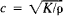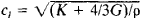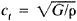# Elastic Wave

Also found in: Wikipedia.

## elastic wave

[i′las·tik ′wāv]
(acoustics)
(physics)
A wave propagated by a medium having inertia and elasticity (the existence of forces which tend to restore any part of a medium to its original position), in which displaced particles transfer momentum to adjoining particles, and are themselves restored to their original position.

## Elastic Wave

an elastic disturbance that propagates in a solid, liquid, or gaseous medium. Examples of elastic waves include the waves generated in the earth’s crust during earthquakes and sound waves and ultrasonic waves in liquids and gases. When elastic waves propagate, the energy of elastic deformation is transferred in the absence of a flow of matter, which occurs only in special cases, such as during an acoustic wind. Every harmonic elastic wave is characterized by the amplitude and vibration frequency of the particles of the medium, a wavelength, phase and group velocities, and a law governing the distribution of displacements and stresses over the wave front. A special feature of elastic waves is that their phase and group velocities are independent of the wave amplitude and the wave geometry. An elastic wave may be a plane, spherical, or cylindrical wave.

Only longitudinal, or compression, waves, in which the particles of the medium vibrate in the direction of wave propagation, can propagate in liquids and gases, which are elastic with respect to volume but not with respect to shape. The phase velocity of waves in fluids and gases is equal to, where K is the bulk modulus and p is the density of the medium. Sound waves are an example of such elastic waves (see).

Only two types of elastic waves—longitudinal and shear, or transverse, waves—can propagate in a homogeneous and isotrópic solid medium of infinite extent. In longitudinal waves, the particle motion is parallel to the direction of wave propagation, and the deformation is a combination of uniform compression or extension and pure shear. In shear waves, the particle motion is perpendicular to the direction of wave propagation, and the deformation is pure shear. For isotropic solids in bulk form, the phase velocity of longitudinal waves is, and the phase velocity of shear waves is, where G is the modulus of elasticity in shear. Surface waves, which are generally called Rayleigh waves, can propagate at the boundary between a solid half space and a vacuum, liquid, or gas; such waves are a combination of nonuniform longitudinal and shear waves whose amplitudes decrease exponentially with distance from the boundary.

Normal waves propagate in solids with sharply defined boundaries, such as a plate or a rod; such solids are acoustic wave guides. Every normal wave is a combination of several longitudinal and shear waves that propagate at acute angles to the waveguide axis and satisfy, in the aggregate, the following boundary conditions: the absence of mechanical stresses at the wave-guide surface. The number of normal waves in a plate or rod is determined by the thickness or diameter d of the plate or rod, the frequency of the normal waves f, and the medium’s moduli of elasticity. As fd increases, the number n of normal waves that may exist in the wave guide also increases; as fd →∞, n→∞. Normal waves propagate with a velocity dispersion (seeDISPERSION OF SOUND); that is, as fd varies from its critical values to infinity, the phase velocities of the normal waves, as a rule, decrease from ∞ to c, but the group velocities increase from 0 to c,. The distribution of displacements and stresses in the wave over the cross section of the wave guide also depends strongly on the value of fd.

The following two types of normal waves exist in an infinite plate: Lamb waves and transverse normal waves. A plane Lamb wave is characterized by two displacement components, one of which is parallel to the direction of wave propagation and the other is perpendicular to the surfaces of the plate. Depending on the distribution of the displacements relative to the midplane of the plate, Lamb waves may be symmetric or antisymmetric. A particular case of a symmetric Lamb wave is a longitudinal wave in a plate, and a particular case of an antisymmetric Lamb wave is a flexural wave. In a plane transverse normal wave, the displacements are both parallel to the surfaces of the plate and perpendicular to the direction of wave propagation. The simplest type of such a wave is a zero-order normal wave, in which the displacements are identical at all points of the plate’s cross section.

Normal waves of the longitudinal, flexural, and torsional types may propagate in cylindrical rods. If the thickness of the rod is small in comparison with the wavelength, then only one normal wave of each type can propagate in the rod.

In anisotropic media, that is, in crystals, the properties of elastic waves and the possibility that such waves exist depend on the type of crystal and the direction of propagation. In particular, purely longitudinal and purely shear waves can propagate only in crystals with specific symmetries (seeSYMMETRY OF CRYSTALS) and only in specific directions, which—as a rule—coincide with the direction of the crystallographic axes. In the general case, elastic waves with three different velocities always propagate in any direction in a crystal; one is a quasi-longitudinal wave, and the other two are quasi-transverse waves in which longitudinal or transverse displacements, respectively, predominate.

Because of the thermal conductivity and the viscosity or internal friction of a medium, the propagation of elastic waves is accompanied by wave damping with distance (seeABSORPTION OF SOUND). If some obstacle, such as a reflecting wall or a vacuum gap, is located on the trajectory of elastic waves, the waves are diffracted by the obstacle. A particular case of diffraction is the reflection and refraction of elastic waves at a plane boundary between two half spaces.

In elastic waves, the stresses are proportional to the strains; that is, Hooke’s law is satisfied. If the strain amplitude in a wave is so great that the stress exceeds the elastic limit of a substance, plastic deformation occurs as the wave passes through the substance, and the wave is said to be elastoplastic. In a liquid or gas, the same type of wave is called a finite-amplitude wave.

### REFERENCES

Landau, L. D., and E. M. Lifshits. Teoriia uprugosti, 3rd ed. Moscow, 1965. (Teoretich. fizika, vol. 7.)
Kolsky, H. Volny napriazheniia v tverdykh telakh. Moscow, 1955. (Translated from English.)
Morse, P. Kolebaniia i zvuk. Moscow-Leningrad, 1949. (Translated from English.)
Brekhovskikh, L. M. Volny v sloistykh sredakh, 2nd ed. Moscow, 1973.
Viktorov, I. A. Fizicheskie osnovy primeneniia ul’trazvukovykh voln Releia i Lemba v tekhnike. Moscow, 1966.

I. A. VIKTOROV

References in periodicals archive ?
Periodic loading on the structure causes its dynamic response as an elastic wave. Guided waves can be widely applied in various fields such as non-destructive testing (NDT) .
where [[phi].sub.2](x, t), [[phi].sub.2](x, t)--the known at cavity boundary of function, determined by incident elastic wave potential ;
The elastic wave velocity of rock is mainly affected by rock type, density, fissure or interlayer, and water content.
Elastic wave propagation in solids produces small displacements [bar.U]=u[bar.i] + v[bar.j] + w[bar.k], which depend on position, producing thus strain and according to the constitutive equations of the material, generate mechanical stresses.
Section 4 presents two benchmark problems, namely, the elastic deformation of a simple supported beam and the elastic wave propagation of a 2D rod, to study the sources of instabilities and the effect of present instability control method.
Gardner, "An experimental investigation of factors affecting elastic wave velocities in porous media," Geophysics, vol.
Batzle, "Changes in elastic wave velocity and rock microstructure due to basalt-C[O.sub.2]-water reactions," Journal of Geophysical Research: Solid Earth, vol.
To detect internal damage, the elastic wave tomography and AE tomography  are under development.
indicate the effect of transversely polarized elastic wave on thermoelastic effect, whereas the effect of thermoelasticity on elastic shear wave having X-polarization is described by [b.sub.47], while the effect of thermoelasticity on transverse polarized wave is given by [b.sub.67].
Each snap provides a discrete pulse of energy that propagates throughout the surrounding material in the form of a transient elastic wave. The frequency content of these pulse-like transient waves is broadband, ranging from a few KHz to a few MHz.
In this section, the five steps for developing the ROM are presented: (1) definition of local coordinate systems, (2) elastic wave propagation in spectral domain, (3) scattering phenomenon and boundary conditions, (4) phase matrix and reverberation matrix generation, and (5) dynamic response calculation.
where c is the elastic wave speed, A,[??],B,C, and D are material parameters, F(u) and G([[phi].sub.x]) are nonlinear contributions at macro- and microscale, respectively, a and b are scaling coefficients.

Site: Follow: Share:
Open / Close